# SAT Math Multiple Choice Question 12: Answer and Explanation

### Test Information

Question: 12

2. For the equation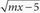= x + 3, the value of m is -3. What is the solution set for the equation?

• A. {-3, 3}
• B. {-2}
• C. {-2, -7}
• D. {3, 6}

B Since the question gives the value of m, the first step is to plug that value into the original equation to get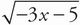= x + 3. Now square both sides of the equation to remove the square root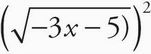= (x + 3)2: or -3x - 5 = x2 + 6x + 9. Now combine like terms. If you combine the terms on the right side of the equation, you can avoid having a negative x2 term. The equation becomes 0 = x2 + 9x + 14. Factor the equation to find the roots: 0 = (x + 2)(x + 7). The possible solutions to the quadratic are -2 and -7. Don't forget to plug these numbers back into the original equation to check for extraneous solutions. Begin by checking x = -2. When you do this, you get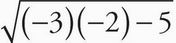= (-2) + 3, or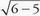= 1, or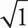= 1, which is true. Now, check x = -7. Set it up as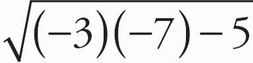= (-7) + 3, and start simplifying to get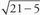= -4. You can technically stop simplifying here, as there is a negative number on the right-hand side of the equal sign. Remember, when taking a square root with a radical provided, it will yield the positive root only. So -7 cannot be part of the solution set. Be very careful of trap answer (C), and select (B).